Question

Find the speed for a star in which this line appears at wavelength 120.2 nm . Express your answer to three significant figures and include the appropriate units. v

1.minhkhue

The speed of the star is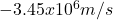Explanation:

Spectral lines will be shifted to the blue part of the spectrum if the source of the observed light is moving toward the observer, or to the red part of the spectrum when it is moving away from the observer (that is known as the Doppler effect).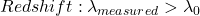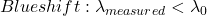The wavelength at rest is 121.6 nm (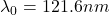)

Due to that shift the velocity of the star can be determined by means of Doppler velocity.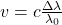(1)

Where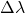is the wavelength shift,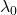is the wavelength at rest, v is the velocity of the source and c is the speed of light.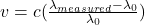In this case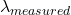is equal to 120.2 nm.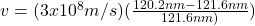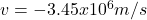Hence, the speed of the star is.

Notice that the negative velocity means that the star is moving toward the observer.